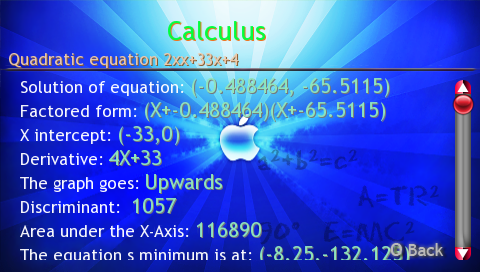This is an educational tool for PSP which “aids” students in areas of Algebra I, Algebra II, Calculus/PreCalculus, Physics I and Physics AP and any type of math with use of inbuilt calculator.It spans a total of 45+ Equations. The usage is simple: user simple inputs the variables for equations/formulas and it does pages of tiresome work automatically and INSTANTLY.

http://www.neoflash.com/forum/index.php/topic,4884.0.html